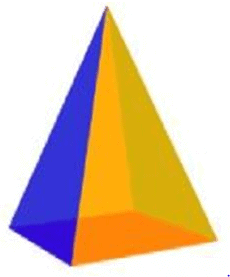# Test: Visualising Solid Shapes- 2

## 20 Questions MCQ Test NCERT Mathematics for CAT Preparation | Test: Visualising Solid Shapes- 2

Description
Attempt Test: Visualising Solid Shapes- 2 | 20 questions in 20 minutes | Mock test for Class 8 preparation | Free important questions MCQ to study NCERT Mathematics for CAT Preparation for Class 8 Exam | Download free PDF with solutions
QUESTION: 1

Solution:
QUESTION: 2

### Find the side of a cube whose surface area is 2400 cm2.

Solution:

Surface area of  a cube = 6l2, where l is the length of its side
2400= 6l2
l= 2400/6
l2  = 400 = 202
l = 20 cm

QUESTION: 3

### Which of the statements is false for a pyramid?

Solution:
QUESTION: 4

Which of the statements is true?

Solution:

A square pyramid is a pyramid with a square base. So it has 4 triangular faces and 1 square base.

QUESTION: 5

Which of the following can be calculated only for a cone but not for a cylinder?

Solution:
QUESTION: 6

Which of the following statements is true?

Solution:
QUESTION: 7

Identify the statement that is false for a prism.

Solution:
QUESTION: 8

Identify the statement that is true.

Solution:
QUESTION: 9

The faces of a square prism consist of

Solution:
QUESTION: 10

A three dimensional shape is _________ object.

Solution:

Solid geometry is the traditional mane of the geometry of three-dimensional

QUESTION: 11

A square pyramid always has ___.

Solution:
QUESTION: 12

The faces of a triangular pyramid consist of

Solution:

There are 4 triangles in a triangular pyramid
A triangular pyramid is a pyramid with a triangle as a base and three triangular faces. It has four vertices or points and six edges.

QUESTION: 13

The faces of a pentagonal pyramid consist of

Solution:
QUESTION: 14

What is the name of this solid?Solution:
QUESTION: 15

Which is the three-dimensional figure formed by rotating a triangle?

Solution:
QUESTION: 16

The faces of a cube consist of

Solution:
QUESTION: 17

How many circular bases does a cylinder have?

Solution:

A cylinder is similar to a prism, but its two bases are circles, not polygons. Also the sides of a cylinder are curved, not flat.
There are 2 circular bases 1 on top and 1 on bottom.

QUESTION: 18

A cylinder has ____.

Solution:
QUESTION: 19

A hexagonal pyramid has ______.

Solution:
QUESTION: 20

An octagonal prism has ____.

Solution:Use Code STAYHOME200 and get INR 200 additional OFF Use Coupon Code